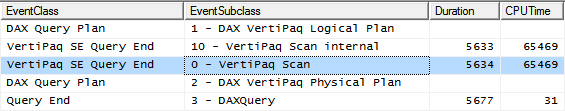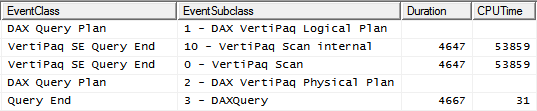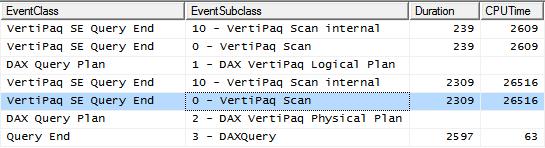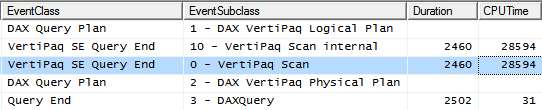With SQL Server Analysis Services (SSAS) 2012 SP1, we welcomed the DIVIDE function, which is handy because it avoids writing complex conditional logic. In fact, instead of writing:

```IF (
SUM ( Sales[SalesAmount] ) <> 0,
SUM ( Sales[GrossMargin] ) / SUM ( Sales[SalesAmount] ),
BLANK ()
)
```

You can now write a much cleaner:

```DIVIDE (
SUM ( Sales[GrossMargin] ),
SUM ( Sales[SalesAmount] ),
BLANK ()
)
```

You avoid writing the code of the numerator twice and, as a plus, DIVIDE is generally faster than the division, avoiding the need to add error handling to your formulas.

Thus, should you dive into all of your existing code and replace any normal division with DIVIDE? As usual, the answer is “it depends”. While it is true that DIVIDE runs faster than a simple division, you need to know that DIVIDE is always executed inside Formula Engine (FE); for this reason, sometimes it can be slower than a simple division. To learn about this, let us start with this query, running on a 4 billion rows table (it is hard to see the difference on smaller datasets):

```EVALUATE
ROW (
"Result",
SUMX (
Audience,
IF (
Audience[Age] <> 0,
Audience[Weight] / Audience[Age]
)
)
)
```

We already know that the presence of the IF statement inside the loop initiated by SUMX will force FE to kick in through a CALLBACKDATAID. Here you can see the trace of the query:The main VertiPaq query (highlighted in the figure) includes a CALLBACKDATAID call, which is required to evaluate the IF statement, and is not available to the VertiPaq Storage Engine (SE):

```SELECT
SUM ( [CallbackDataID ( IF (
Audience[Age]] <> 0,
Audience[Weight]] / Audience[Age]]
) ) ](PFDATAID ( [Audience].[Weight] ), PFDATAID ( [Audience].[Age] )))
FROM [Audience];
```

The overall duration is 5.6 seconds, running in parallel on all cores. But, as you might already know, the presence of FE prevents the usage of the VertiPaq cache. In fact, if we run the query again without clearing the cache, the resulting execution time is still 5.6 seconds.

If we rewrite the query using DIVIDE, in this way:

```EVALUATE
ROW (
"Result",
SUMX (
Audience,
DIVIDE (
Audience[Weight],
Audience[Age]
)
)
)
```

The query plan shows a good improvement: the query runs now in 4.6 seconds, with a gain of 17% obtained by simply changing one function call, which is not bad at all.Nevertheless, the main VertiPaq SE query still contains a CALLBACKDATAID, because DIVIDE is not available in SE, it is an optimized function executed in FE:

```SELECT
SUM ( [CallbackDataID ( DIVIDE (
Audience[Weight]],
Audience[Age]]
))] ( PFDATAID ( [Audience].[Weight] ), PFDATAID ( [Audience].[Age] )))
FROM [Audience];
```

Thus, running the query again leads to 4.6 seconds execution time. Even in this case, we cannot make a good usage of the cache.

We can rewrite the same query using CALCULATE, avoiding any iteration on rows where the denominator equals to zero in this way:

```EVALUATE
ROW (
"Result",
CALCULATE (
SUMX (
Audience,
Audience[Weight] / Audience[Age]
),
Audience[Age] <> 0
)
)
```

Now CALCULATE gets rid of the rows where Age is equal to zero. We use the simple division operator that, unlike CALCULATE, is available in SE. The resulting query plan shows that there are now two distinct SE queries: the first one retrieves the values of Age different than zero, the second one computes the sum of Weight divided by Age. This time, we do not need to check for the denominator, as we already know it will be other than zero.We have been able to reduce the duration to 2.6 seconds (running more than twice as fast as the first query). Moreover, the most interesting part of this second query is the fact that the VertiPaq SE scan shows no CALLBACKDATAID:

```SELECT
SUM (
CAST ( PFCAST( [Audience].[Weight] AS INT ) AS REAL )
/
CAST ( PFCAST( [Audience].[Age] AS INT ) AS REAL )
)
FROM [Audience]
WHERE
[Audience].[Age] IN (68, 97, 4, 49, 54...[96 total values, not all displayed]);
```

This is a pure SE query plan, which can be cached by the engine. In fact, running it the second time, we get an execution time of 3 milliseconds: just the time needed to retrieve the values from the cache.

Striving for perfection, you can try this last formulation of the query:

```EVALUATE
ROW (
"Result",
SUMX (
FILTER ( Audience, Audience[Age] <> 0 ),
Audience[Weight] / Audience[Age]
)
)
```

This time, instead of using IF logic or CALCULATE to remove zeroes, we used a FILTER statement and iterate over its result. The resulting query plans shows a single SE query:The execution time is reduced to 2.5 seconds. The single VertiPaq SE Query contains:

```SELECT
SUM (
CAST ( PFCAST( [Audience].[Weight] AS INT ) AS REAL )
/
CAST ( PFCAST( [Audience].[Age] AS INT ) AS REAL )
)
FROM [Audience]
WHERE
(PFDATAID( [Audience].[Age] ) <> 2);
```

You can see that now the filtering of Age <> 0 is injected in the single VertiPaq query that computes the result. The gain, in this example, is tiny because the number of distinct values of the Age column is very small (near a 100 values) and the column is very well compressed. For columns with a larger number of distinct values, the improvement might be much more. As always, DAX performance strongly depend on the cardinality and distribution of values. Even this last query, of course, is fully cached because it uses only SE.

As you have seen, DIVIDE normally provides better performance than the division operator. That said, DIVIDE always forces FE to evaluate the expression and this, in many scenarios, leads to poor cache usage and worse performance. A careful investigation of the query plan and the simple rewriting of the query to force a pure SE query leads to a better cache usage and to optimal performances. This technique might not work for more complex formulas and data models, so always test your specific scenario to verify which approach works better for your data.

DIVIDESafe Divide function with ability to handle divide by zero case.

`DIVIDE ( <Numerator>, <Denominator> [, <AlternateResult>] )`

IFChecks whether a condition is met, and returns one value if TRUE, and another value if FALSE.

`IF ( <LogicalTest>, <ResultIfTrue> [, <ResultIfFalse>] )`

SUMXReturns the sum of an expression evaluated for each row in a table.

`SUMX ( <Table>, <Expression> )`

CALCULATEContext transition

Evaluates an expression in a context modified by filters.

`CALCULATE ( <Expression> [, <Filter> [, <Filter> [, … ] ] ] )`

FILTERReturns a table that has been filtered.

`FILTER ( <Table>, <FilterExpression> )`